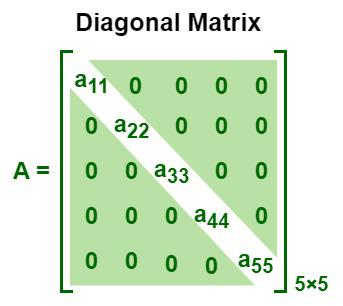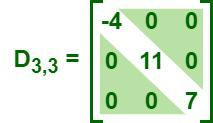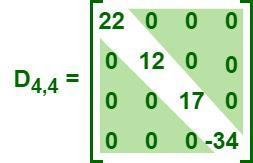Open in App
Not now

# Diagonal Matrix

• Last Updated : 09 Jan, 2023

A matrix is defined as a rectangular array of numbers that are arranged in rows and columns. The size of a matrix can be determined by the number of rows and columns in it. A matrix is said to be an “m by n” matrix when it has “m” rows and “n” columns and is written as an “m × n” matrix. For example, the order of the matrix that has five rows and four columns is “5 × 4.” We have different types of matrices, such as rectangular, square, triangular, symmetric, singular, etc. The image given below is an “m × n” matrix that has “m” rows and “n” columns.

## What is a Diagonal Matrix?

A diagonal matrix is a square matrix in which all of the elements except the principal diagonal elements are zeroes. It is both upper and lower triangular, as all the elements except the main diagonal elements are zeros. A square matrix “A = [aij]” is said to be a diagonal matrix if aij = 0 when i ≠ j. The matrix given below represents a diagonal matrix of order “5 × 5.”## Examples of a Diagonal Matrix

Some common examples of diagonal matrices of different orders are given below:

• Diagonal Matrix of order (3 × 3)• Diagonal Matrix of order (4 × 4)## Properties of a Diagonal Matrix

The following are the properties of the Singular Matrix:

• Every diagonal matrix is a square matrix, i.e., a matrix that has an equal number of rows and columns.
• Scalar matrices, identity matrices, and null matrices are examples of diagonal matrices, as their non-principal diagonal elements are zeros.
• The resultant matrix of the sum of two diagonal matrices is also a diagonal matrix.
• The resultant matrix of the product of diagonal matrices is also a diagonal matrix, where the main diagonal elements of the resultant matrix are products of the corresponding elements of the original matrices.

Ifand, then.

• A diagonal matrix is a symmetric matrix, as the transpose of a diagonal matrix is the same matrix itself.

Ifis a diagonal matrix, then, i.e., D = DT.

• A diagonal matrix is a symmetric matrix, as the transpose of a diagonal matrix is the same matrix itself.

Ifandare two diagonal matrices, then## Block Diagonal Matrix

The term “block matrix” refers to a matrix that is divided into blocks. In such matrices, the non-diagonal blocks are zero matrices, whereas the main diagonal blocks are square matrices. A matrix “A = [aij]” is called a block diagonal matrix when aij = 0, for i ≠ j, i.e., when the non-diagonal blocks are zero.## Determinant of a Diagonal Matrix

The determinant of a diagonal matrix is equal to the product of its principal diagonal elements.

If,|D| = a11 × a22 × a33.

## Inverse of a Diagonal Matrix

The inverse of a diagonal matrix is also a diagonal matrix with the principal diagonal’s elements being the reciprocals of the corresponding elements of the original matrix.

If, then.

## Anti-Diagonal Matrix

An anti-diagonal matrix, or non-diagonal matrix, is said to be the mirror image of a diagonal matrix concerning the placement of the elements. In an anti-diagonal matrix, all elements are zero except the diagonal (not the principal diagonal) elements from the upper right edge to the lower left edge. The matrix given below is an anti-diagonal matrix of order “3 × 3.”Also, Check

## Solved Examples on Diagonal Matrix

Example 1: If, then prove that A = AT.

Solution:

Given matrix, A == A

So, A = AT

Hence proved.

Example 2: Find the determinant of the matrix given below.Solution:

Given matrix, D =We can notice that the given matrix is a diagonal matrix. We know that the determinant of a diagonal matrix is equal to the product of its principal diagonal elements.

So, |D| = -5 × 0 × 14 = 0

Hence, the determinant of the given matrix is 0.

Example 3: Find the inverse of the matrix given below.Solution:

We can notice that the given matrix is a diagonal matrix. We know that the inverse of a diagonal matrix is obtained by replacing the principal diagonal’s elements with the reciprocals of the corresponding elements of the original matrix, while the rest of the elements remain the same.

So,.

Example 4: Prove that A + B = B + A, ifand.

Solution:

Given matrices,So, A + B = B + A

Hence, proved.

## FAQs on Diagonal Matrix

### Question 1: Define a diagonal matrix.

A diagonal matrix is a square matrix in which all of the elements except the principal diagonal elements are zeroes.

### Question 2: Is an identity matrix a diagonal matrix?

An identity matrix is a square matrix whose principal diagonal elements are ones and the rest of the elements are zeros. So, an identity matrix or unit matrix is a diagonal matrix.

### Question 3: What is the determinant of a diagonal matrix?

The determinant of a diagonal matrix is equal to the product of its principal diagonal elements.

If, then |D| = a11 × a22 × a33.